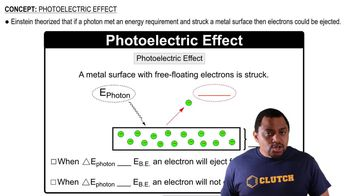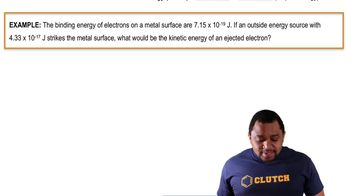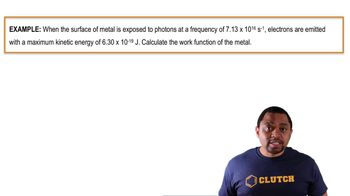Start typing, then use the up and down arrows to select an option from the list.# Photoelectric Effect

Jules Bruno
220views
1
so we know that if enough energy is bombarded on the metal surface, it'll kick in. Electron off. Now we use the brief abbreviation be this stands for binding energy here. This is the minimum amount of energy needed to inject an electron from a metal surface. We're gonna say it's also known as our threshold frequency or our work function of the metal. So it goes by a lot of different names. So binding energy, threshold frequency as well as work function now kinetic energy. We say that this is the energy and elect object has due to its speed or motion. So basically any excess energy that the electron games from this light source will be converted into kinetic energy. Now, from this, we can say that the photo electric formula photo electric effect formula is total energy, which is the photon equals the binding energy, plus your kinetic energy, which we say is your surplus energy. So when we're dealing with any type of photo electric question that requires some type of calculation, it usually deals with this formula. So total energy equals binding energy plus kinetic energy01:2601:1101:2201:1801:52